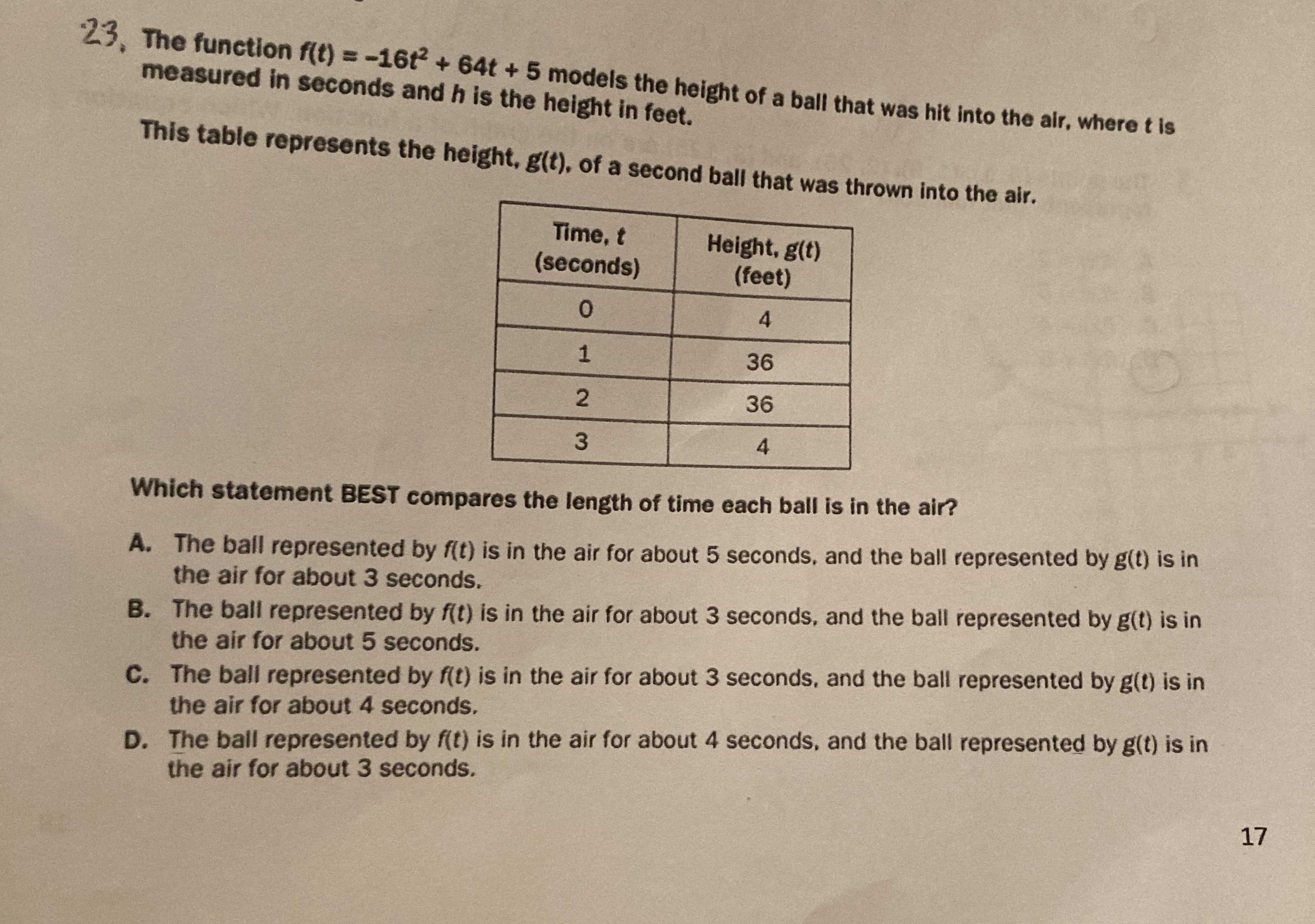### ¿Todavía tienes preguntas de matemáticas?

Pregunte a nuestros tutores expertos
Algebra
Pregunta23. The function $$f ( t ) = - 16 t ^ { 2 } + 64 t + 5$$ models the height of a ball that was hit into the air, where $$t$$ is measured in seconds and $$h$$ is the height in feet. This table represents the height, $$g ( t )$$ , of a second ball that was thrown into the air. Which statement BEST compares the length of time each ball is in the air? A. The ball represented by $$f ( t )$$ is in the air for about $$5$$ seconds, and the ball represented by $$g ( t )$$ is in the air for about $$3$$ seconds. B. The ball represented by $$f ( t )$$ is in the air for about $$3$$ seconds, and the ball represented by $$g ( t )$$ is in the air for about $$5$$ seconds. C. The ball represented by $$f ( t )$$ is in the air for about $$3$$ seconds, and the ball represented by $$g ( t )$$ is in the air for about $$4$$ seconds. D. The ball represented by $$f ( t )$$ is in the air for about $$4$$ seconds, and the ball represented by $$g ( t )$$ is in the air for about $$3$$ seconds.